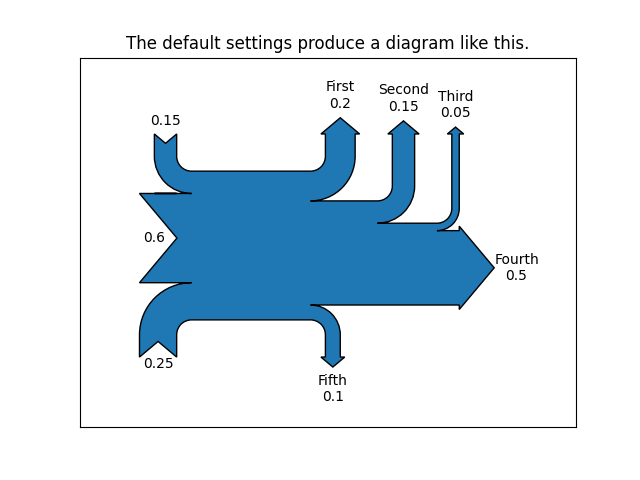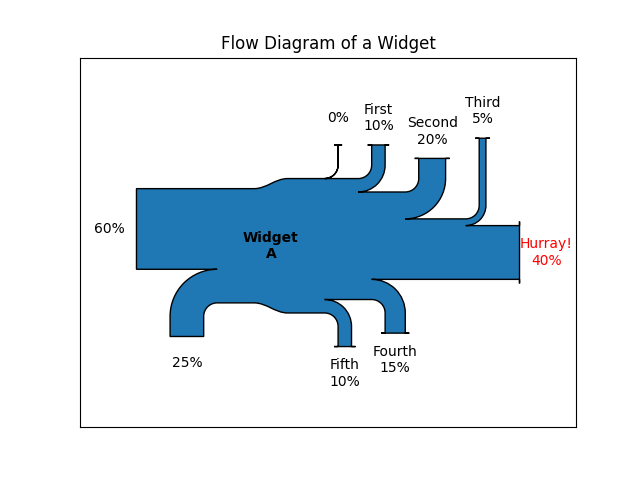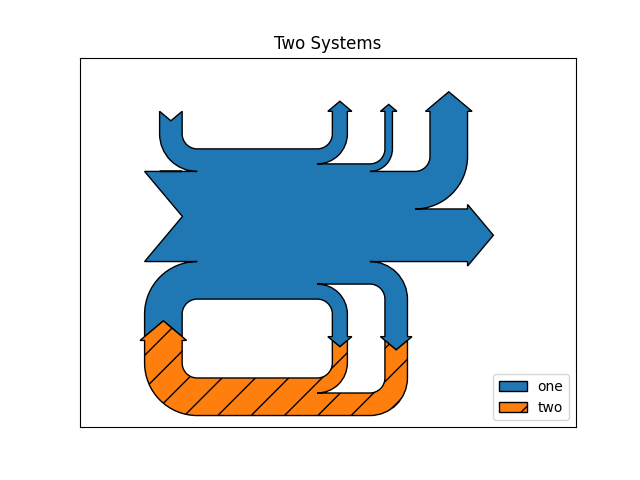# The Sankey class¶

Demonstrate the Sankey class by producing three basic diagrams.

import matplotlib.pyplot as plt

from matplotlib.sankey import Sankey


Example 1 -- Mostly defaults

This demonstrates how to create a simple diagram by implicitly calling the Sankey.add() method and by appending finish() to the call to the class.

Sankey(flows=[0.25, 0.15, 0.60, -0.20, -0.15, -0.05, -0.50, -0.10],
labels=['', '', '', 'First', 'Second', 'Third', 'Fourth', 'Fifth'],
orientations=[-1, 1, 0, 1, 1, 1, 0, -1]).finish()
plt.title("The default settings produce a diagram like this.")Out:

Text(0.5, 1.0, 'The default settings produce a diagram like this.')


Notice:

1. Axes weren't provided when Sankey() was instantiated, so they were created automatically.
2. The scale argument wasn't necessary since the data was already normalized.
3. By default, the lengths of the paths are justified.

Example 2

This demonstrates:

1. Setting one path longer than the others
2. Placing a label in the middle of the diagram
3. Using the scale argument to normalize the flows
4. Implicitly passing keyword arguments to PathPatch()
5. Changing the angle of the arrow heads
6. Changing the offset between the tips of the paths and their labels
7. Formatting the numbers in the path labels and the associated unit
8. Changing the appearance of the patch and the labels after the figure is created
fig = plt.figure()
ax = fig.add_subplot(1, 1, 1, xticks=[], yticks=[],
title="Flow Diagram of a Widget")
sankey = Sankey(ax=ax, scale=0.01, offset=0.2, head_angle=180,
format='%.0f', unit='%')
sankey.add(flows=[25, 0, 60, -10, -20, -5, -15, -10, -40],
labels=['', '', '', 'First', 'Second', 'Third', 'Fourth',
'Fifth', 'Hurray!'],
orientations=[-1, 1, 0, 1, 1, 1, -1, -1, 0],
pathlengths=[0.25, 0.25, 0.25, 0.25, 0.25, 0.6, 0.25, 0.25,
0.25],
patchlabel="Widget\nA")  # Arguments to matplotlib.patches.PathPatch
diagrams = sankey.finish()
diagrams.texts[-1].set_color('r')
diagrams.text.set_fontweight('bold')Notice:

1. Since the sum of the flows is nonzero, the width of the trunk isn't uniform. The matplotlib logging system logs this at the DEBUG level.
2. The second flow doesn't appear because its value is zero. Again, this is logged at the DEBUG level.

Example 3

This demonstrates:

1. Connecting two systems
2. Turning off the labels of the quantities
fig = plt.figure()
ax = fig.add_subplot(1, 1, 1, xticks=[], yticks=[], title="Two Systems")
flows = [0.25, 0.15, 0.60, -0.10, -0.05, -0.25, -0.15, -0.10, -0.35]
sankey = Sankey(ax=ax, unit=None)
orientations=[-1, 1, 0, 1, 1, 1, -1, -1, 0])
orientations=[-1, -1, -1], prior=0, connect=(0, 0))
diagrams = sankey.finish()
diagrams[-1].patch.set_hatch('/')
plt.legend()Out:

<matplotlib.legend.Legend object at 0x7f27da3bd550>


Notice that only one connection is specified, but the systems form a circuit since: (1) the lengths of the paths are justified and (2) the orientation and ordering of the flows is mirrored.

plt.show()